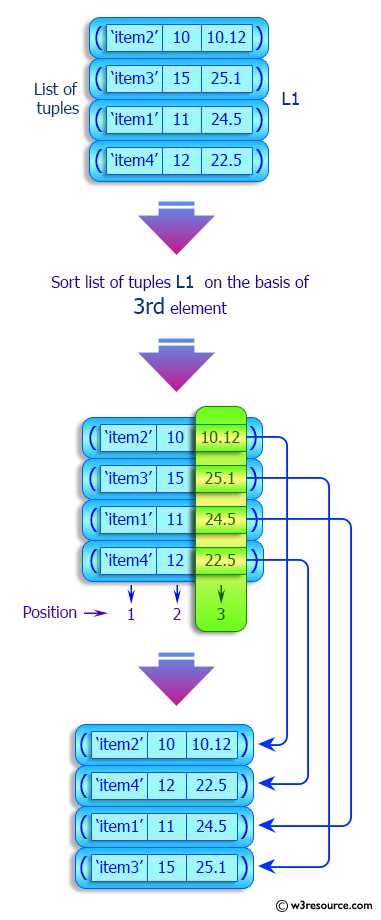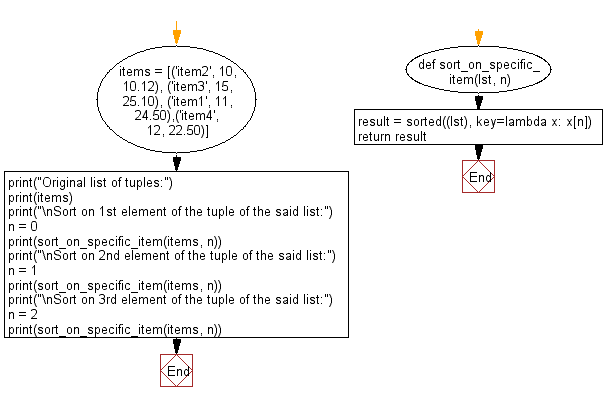﻿ Python: Sort a given list of tuples on specified element - w3resource

# Python: Sort a given list of tuples on specified element

## Python List: Exercise - 188 with Solution

Write a Python program to sort a given list of tuples by a specified element.

Sample Solution:

Python Code:

``````def sort_on_specific_item(lst, n):
result = sorted((lst), key=lambda x: x[n])
return result
items = [('item2', 10, 10.12), ('item3', 15, 25.10), ('item1', 11, 24.50),('item4', 12, 22.50)]
print("Original list of tuples:")
print(items)
print("\nSort on 1st element of the tuple of the said list:")
n = 0
print(sort_on_specific_item(items, n))
print("\nSort on 2nd element of the tuple of the said list:")
n = 1
print(sort_on_specific_item(items, n))
print("\nSort on 3rd element of the tuple of the said list:")
n = 2
print(sort_on_specific_item(items, n))
```
```

Sample Output:

```Original list of tuples:
[('item2', 10, 10.12), ('item3', 15, 25.1), ('item1', 11, 24.5), ('item4', 12, 22.5)]

Sort on 1st element of the tuple of the said list:
[('item1', 11, 24.5), ('item2', 10, 10.12), ('item3', 15, 25.1), ('item4', 12, 22.5)]

Sort on 2nd element of the tuple of the said list:
[('item2', 10, 10.12), ('item1', 11, 24.5), ('item4', 12, 22.5), ('item3', 15, 25.1)]

Sort on 3rd element of the tuple of the said list:
[('item2', 10, 10.12), ('item4', 12, 22.5), ('item1', 11, 24.5), ('item3', 15, 25.1)]
```

Pictorial Presentation:Flowchart:## Visualize Python code execution:

The following tool visualize what the computer is doing step-by-step as it executes the said program:

Python Code Editor:

Have another way to solve this solution? Contribute your code (and comments) through Disqus.

What is the difficulty level of this exercise?

Test your Programming skills with w3resource's quiz.

﻿

## Python: Tips of the Day

Decapitalizes the first letter of a string:

Example:

```def tips_decapitalize(s, upper_rest=False):
return s[:1].lower() + (s[1:].upper() if upper_rest else s[1:])
print(tips_decapitalize('PythonTips'))
print(tips_decapitalize('PythonTips', True))
```

Output:

```pythonTips
pYTHONTIPS
```

We are closing our Disqus commenting system for some maintenanace issues. You may write to us at reach[at]yahoo[dot]com or visit us at Facebook